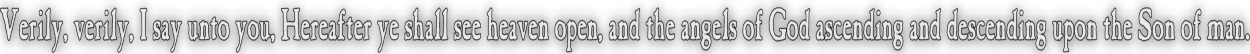None:
Polyps:
Strongs:CreationismLogical OR The analogy to logical OR takes some time to show. d = 1 = {e,f,g} opposes the three balances with d as wine ; {d,f,g} => {d,e,g} <= {d,e,f} (f as oil) {d,f,g} => {d,e,f} <= {d,e,g} (g as oil) {d,e,g} => {d,f,g} <= {d,e,f} (e as oil) Likewise with d = 1 = {e,f,g} as oil, we have with choices of wine {d,e,f} => {e,f,g} <= {d,e,g} (e as wine) {d,e,f} => {e,f,g} <= {d,f,g} (f as wine) {d,e,g} => {e,f,g} <= {d,f,g} (g as wine) We note that each row has its pairing of "negatives" of syntheses such as; {d,f,g} => {d,e,f} <= {d,e,g} (g as oil, d as wine) {d,e,g} => {e,f,g} <= {d,f,g} (d as oil, g as wine) These two (syntheses) as negatives of each other may become a new thesis/antithesis {e,f,g} => {d,e,g} <= {d,e,f} (f as oil, e as wine) {e,f,g} => {d,f,g} <= {d,e,f} (e as oil, f as wine) Now, the synthesis of these last two are the same thesis/antithesis of our original pairing of rows above!. So, in the same manner that our disjunction becomes our conjunction, we have the result that the syntheses of opposites are the opposites from which the synthesis of our opposing premises arise. That is; A&B and C&D the syntheses or conjunction/disjunction of A v B with C&D = ~(A&B) ('~' is as our new "dialectic" NOT) leads to C&D v A&B has the syntheses X and ~X, where X is actually either A or B. So how does this equate to a logical OR? Simply that in each case the statement is such as to show there is some appearance of "common ground" - we actually have some idea that there will always be an excluded middle, but we may happily recover in the process our original thesis/antithesis pair - the information is "not lost" in the process, which leads to some idea of negation of negation becoming an action of identity. The elements of oil and wine that are "inverse" to each other are divided so that A v B related to {d,g} as oil/wine and C&D v A&B relates to {e,f} as oil/wine. It is the division of {d,e,f,g} into two pairs that divides each side of the logical "OR" statement's analogy in the dialectic. Since the "opposites" of A and B (or AvB and CvD) always "have their conjunction", the division of oil and wine into two disjoint sets has the result that two totally and irreconcilable opposing views have their conjunction in the method itself only. We actually have as an "OR" statement "chicken" OR "egg", and an endless argument without resolution unless one party compromises. In this sense the dialectic method is the only synthesis, and we may write our logical "OR" statement. Continue To Next Page Return To Section Start Return To Previous Page'